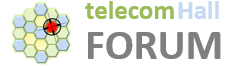# MIMO: MCS in different layers

Hello,

Can someone help with the following questions?

1. If we have 2 or 4 layer MIMO then a code block (or the transport block) is sent across multiple layers. Correct? Or is each layer used for one CBG/TBS?

2. If digital beamforming is used, then each layer would see a different BF gain (based on the eigenvalues/eigenvectors of the H matrix). Therefore the channel quality on each layer would be different. Now assuming the code block can be sent across multiple layers, will the code block use different MCS or the same MCS in each of the layers?

Answer 1 : max 2 TB can be transmitted irrespective of number of layers. 2 TB can be used with minimum 2 layers Example : with 2 layers ,if it is 1 TB only then symbols will be distributed equally on both layer.

Answer 2 : MCS is decided for each TB, hence 2 TB can have different MCS. Number of layers is decided on csi feedback from UE , and CSI feedback is not layer specific but for overall channel. Using above example , both layers will carry symbols from same TB , hence same MCS.

Thank you @needoos.th

Can you please let me know how is TB defined when (i) code block segmentation is used and (ii) under MU-MIMO operation?

My view is, different MCS for different layer.

It is important to note concept of MIMO layers with beamforming.

With beamforming and MIMO, you have two fold layers:

1. Beamforming layers, so different users being served in same TTI using same subcarriers
2. MIMO layers for each of those MU-MIMO users, can be 2x1, 2x2.

So

• MCS across MIMO layers will be same for each user.
• MCS across beamforming layers can be different depending on individual users own conditions.

MCS across MIMO layers for same user can be different if two codewords are getting transmitted.

Only relationship between Code block segmentation and TB is the size. If TB is bigger then x bits , then multiple code blocks will be created.

With MuMimo , for TB size only important aspect is number of layers for a single user. If there are two layers , then capacity is approx. double , hence TB size is bigger.